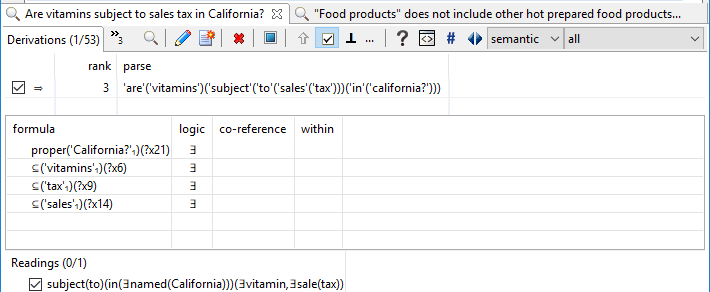# generalized quantifiers

## Is business logic too much for classical logic?

Business logic is not limited to mathematical logic, as in first-order predicate calculus.

Business logic commonly requires “aggregation” over sets of things, like summing the value of claims against a property to subtract it from the value of that property in order to determine the equity of the owner of that property.

• The equity of the owner of a property in the property is the excess of the value of the property over the value of claims against it.

There are various ways of describing such extended forms of classical logic.  The most relevant to most enterprises is the relational algebra perspective, which is the base for relational databases and SQL.  Another is the notion of generalized quantifiers.

In either case, it is a practical matter to be able to capture such logic in a rigorous manner.  The example below shows how that can be accomplished using English, producing the following axiom in extended logic:

• ∀(?x15)(property(?x15)→∀(?x10)(owner(of)(?x10,?x15)→∃(?x31)(value(of(?x15))(?x31)∧∑(?x44)(∀(?x49)(claim(against(?x15))(?x49)→value(of(?x49))(?x44))→∃(?x26)(excess(of(?x31))(over(?x44))(?x26)∧equity(in(?x15))(of(?x10))(?x26))))))

This logic can be realized in various ways, depending on the deployment platform, such as: (more…)